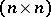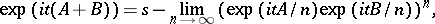# Trotter product formula

(diff) ← Older revision | Latest revision (diff) | Newer revision → (diff)

The following formula for the exponential of two not necessarily commuting operators:(a1)

The easiest case of this is to see this as a (formal) identity in the completion (with respect to the augmentation ideal) of the free associative algebra overin the variablesand, where bothandare given degree.

The case of (a1) whereandare-matrices is due to S. Lie, and is simply known as the product formula for matrix exponentials.

In the formwhich is important in theoretical physics, it holds, e.g., whenandare self-adjoint operators on a separable Hilbert space such that, defined on the intersection of the domains ofand, is essentially self-adjoint. And in the formit holds (for positive) ifandare bounded from below.

Collectively these results (and several more variants) are also known as the Trotter–Kato theorem.

The Trotter product formula finds many applications in quantum theory, both in theoretical and in simulation studies (of quantum spin systems, e.g).

How to Cite This Entry:
Trotter product formula. Encyclopedia of Mathematics. URL: http://encyclopediaofmath.org/index.php?title=Trotter_product_formula&oldid=19177